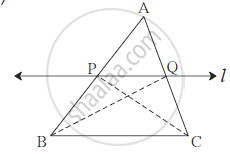Advertisement Remove all ads

# Prove that : “If a Line Parallel to a Side of a Triangle Intersects the Remaining Sides in Two Distince Points, Then the Line Divides the Sides in the Same Proportion.” - Geometry

Prove that :
“If a line parallel to a side of a triangle intersects the remaining sides in two distince points, then the line divides the sides in the same proportion.”

Advertisement Remove all ads

#### SolutionGiven :In ΔABC line l || Side BC line l intersects side AB and side AC in P and Q respectively.
To prove : (AP)/(PB) = (AQ)/(QC)
Construction : Draw seg PC and seg QB.

Proof :  (A(APQ))/(A(PQB)) = (AP)/(PB) ...... (I) (Areas are in proportion to the bases) (A(APQ))/(A(PQB)) = (AQ)/(QC) ....... (II) (Areas are in proportion to the bases) Δ PQB and Δ PQC have the same base PQ and PQ || BC,
their height is also same.
∴ A(Δ PQB) = A(Δ PQC) ..... (III)
∴ (A(APQ))/(A(PQB)) =( A(APQ))/(A(PQC)) ........ from ((I), (II) and (III)
∴ (AP)/(PB) = (AQ)/(QC) ........ from (I) , (II)

Concept: General Equation of a Line
Is there an error in this question or solution?
Advertisement Remove all ads

#### Video TutorialsVIEW ALL 

Advertisement Remove all ads
Share
Notifications

View all notifications

Forgot password?